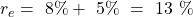Cost of Capital | IFT World
IFT Notes for Level I CFA® Program
IFT Notes for Level I CFA® Program

# Part 2

## 3. Costs of the Various Sources of Capital

Each source of capital has a different cost because of differences in seniority, contractual commitments, and potential value as a tax shield. Three primary sources of capital are:

• Debt
• Preferred equity
• Common equity

### Cost of Debt

Cost of debt is the cost of financing to a company using debt instruments such as taking a bank loan or issuing a bond. In simpler terms, it is the effective interest rate a company pays on its current debt. Two methods to estimate the before-tax cost of debt are:

• The yield to maturity (YTM) approach
• Debt-rating approach

Yield to Maturity Approach

YTM is the annual return an investor earns if the bond is purchased today and held until maturity. It is the rate at which the present value of all future cash flows equals the market price of the bond.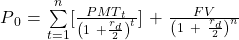where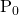= the current market price of the bond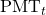= interest payment in period t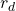= the yield to maturity
n = number of periods remaining to maturity
FV = maturity value of the bond

Example

A company issues a 10-year, 8% semi-annual coupon bond. Upon issuance, the bond sells for $980. If the marginal tax rate is 30%, what is the after-tax cost of debt? Solution: First, calculate the before-tax cost of debt by entering the following values: N = 20 because it is a semi-annual coupon bond, so there are 10 x 2 = 20 periods. PV = -980; the price at which the bond is current selling FV = 1000; the face value of the bond that will be repaid at maturity (the face value of the bond is not explicitly given but assume it is the nearest round figure.) PMT = (0.08/2) * 1000 = 40 (Coupons are always paid on the face value) Compute I/Y = 4.15 % Annual I/Y = 4.15 x 2 = 8.30 = before-tax cost of debt After-tax cost of debt = 8.30 (1 – 0.3) = 5.8% Debt Rating Approach This method is used when the company’s debt doesn’t have an YTM as it is not publicly traded. In such case, the approach is as follows: • Determine the current market rates for comparable bonds with similar ratings and maturities (using matrix pricing). • Analyze the company characteristics like covenants, seniority, etc. to get before-tax cost of debt. • Apply the marginal tax rate to arrive at the after-tax cost of debt. Example A company’s capital structure includes debt with an average maturity of 10 years. The company’s rating is AA, and it has a marginal tax rate of 30%. The yield on comparable AA bonds with similar maturity is 5%. Compute the company’s after-tax cost of debt. Solution: The company’s after-tax cost of debt is: rd(1 – t) = 5%(1 – 0.3) = 3.5% ### Cost of Preferred Stock The cost of preferred stock is the cost that a company has committed to pay to preferred stockholders in the form of preferred dividend. Preferred stock has the characteristics of both debt and equity. Unlike common dividend which is variable, preferred dividend is usually fixed and paid before common shareholders. The cost of preferred stock can be computed as: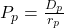where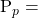the current preferred stock price per share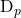= the preferred stock dividend per share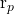= the cost of preferred stock Example A company issues preferred stock with par value$100 that is currently valued at $125 per share. The preferred dividend is$5 per share. The marginal tax rate is 33 percent. What is the cost of preferred stock?

Solution:

Cost of preferred stock = 5/125 = 4%

Note: We ignore taxes, because unlike interest payments, dividends are not tax deductible.

### Cost of Common Equity

Cost of common equity, or cost of equity, is the rate of return required by a company’s common shareholders. It is the return expected by investors for the risk they undertake. Unlike debt and preferred stock, estimating the cost of equity is challenging because of the uncertain nature of future cash flows.

Two commonly used methods to estimate the cost of equity are:

• Capital asset pricing model
• Bond yield plus risk premium method

Capital Asset Pricing Model (CAPM) Approach

According to this method, the cost of equity is equal to the risk free rate plus a premium for bearing the security’s market risk. The premium is the beta for the security multiplied by the equity risk premium.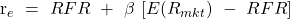where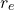= the cost of equity
RFR = risk-free rate of an asset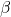= the sensitivity of a stock’s return to changes in market return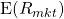= expected return on the marketis also called the equity risk premium because it is the premium that investors expect for investing in the market relative to the risk-free rate.

Example

In a developing market, the risk-free rate is 10% and the equity risk premium is 6%. The equity beta for a given company is 2. What is the cost of equity using the CAPM approach?

Solution:

re = 0.1 + 2 [0.06] = 22%

To estimate the risk-free rate, we use the yields on long-term government bonds. To estimate the equity risk premium we use historical returns. Historical equity risk premium is a good indicator of expected equity risk premium.

Bond Yield plus Risk Premium Method

The bond yield plus risk premium method is commonly used for companies with publicly traded debt. It provides a quick estimate of the cost of equity for such companies.

In this method, we add a risk premium to the yield on the firm’s long-term debt. The assumption here is that the return on a company’s equity will be greater than the return on the company’s bond, as equity is riskier than the bond.

re = bond yield + risk premium

Example

A company’s interest rate on long-term debt is 8%. The risk premium of equity is estimated to be 5%. What is the cost of equity?

Solution: